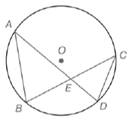Chapter 6.1, Problem 33EElementary Geometry For College St...

7th Edition
Alexander + 2 others
ISBN: 9781337614085

Solutions

Chapter
SectionElementary Geometry For College St...

7th Edition
Alexander + 2 others
ISBN: 9781337614085
Textbook Problem

In Exercises 32 to 37, write a paragraph proof. Given: Chords A B ¯ , B C ¯ , C D ¯ , and A D ¯ in ⊙ O Prove: Δ A B E ∼ Δ C D ETo determine

To Prove: The ABECDE by using the provided figure.

Explanation

Given: The chords AB¯,BC¯,CD¯ and AD¯, in O shown below.

Postulate used:

The measure of an inscribed angles is equal to the one-half the measure of its intercepted arc.

Proof:

Since, A and C both are inscribed angles and BD is their intercepted arcs.

Therefore, there is a theorem state that the measure of an inscribed angles is equal to the one-half the measure of its intercepted arc

Still sussing out bartleby?

Check out a sample textbook solution.

See a sample solution

The Solution to Your Study Problems

Bartleby provides explanations to thousands of textbook problems written by our experts, many with advanced degrees!

Get Started

In Problems 35-38, use the x-intercept method to find one solution of each equation.

Mathematical Applications for the Management, Life, and Social Sciences

Using as an estimator, find the maximum error in estimating with the Midpoint Rule and 8 subintervals. 9

Study Guide for Stewart's Single Variable Calculus: Early Transcendentals, 8th

True or False: If converges and converges, then converges.

Study Guide for Stewart's Multivariable Calculus, 8th